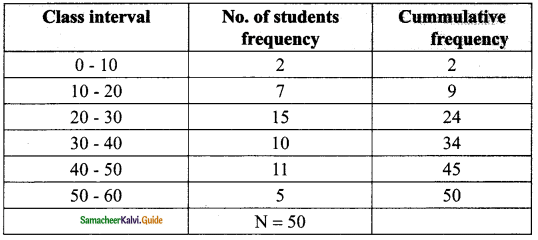Students can download Maths Chapter 8 Statistics Ex 8.2 Questions and Answers, Notes, Samacheer Kalvi 9th Maths Guide Pdf helps you to revise the complete Tamilnadu State Board New Syllabus, helps students complete homework assignments and to score high marks in board exams.

Tamilnadu Samacheer Kalvi 9th Maths Solutions Chapter 8 Statistics Ex 8.2

Question 1.
Find the median of the given values: 47, 53, 62, 71, 83, 21, 43, 47, 41
Solution:
Arrange the values in ascending order we get
21, 41, 43, 47, 47, 53, 62, 71, 83
The number of values = 9 which is odd
Median = ($$\frac{9+1}{2})^{th}$$ variable
= 5th variable
∴ Median = 47Question 2.
Find the median of the given data: 36, 44, 86, 31, 37, 44, 86, 35, 60, 51
Solution:
Arrange the values in ascending order we get
31, 35, 36, 37, 44, 44, 51, 60, 86, 86
The number of values = 10 which is even
Median = Average of ($$\frac{10}{2})^{th}$$ and ($$\frac{10}{2}+1)^{th}$$ value
= Average of 5th and 6th value
= $$\frac{44+44}{2}$$
= $$\frac{88}{2}$$
= 44
∴ Median = 44Question 3.
The median of observation 11, 12, 14, 18, x + 2, x + 4, 30, 32, 35, 41 arranged in ascending order is 24. Find the values of x.
Solution:
The given observation is 11, 12, 14, 18, x + 2, x + 4, 30, 32, 35, 41 (is ascending order)
The number of values =10
Median = Average of ($$\frac{10}{2})^{th}$$ and ($$\frac{10}{2}+1)^{th}$$ value
= Average of 5th and 6th value
24 = $$\frac{x+2.+x+4}{2}$$
24 = $$\frac{2x+6}{2}$$
2x + 6 = 48
2x = 48 – 6
2x = 42
x = $$\frac{42}{2}$$
= 21
The value of x = 21Question 4.
A researcher studying the behavior of mice has recorded the time (in seconds) taken by each mouse to locate its food by considering 13 different mice as 31, 33, 63, 33, 28, 29, 33, 27, 27, 34, 35, 28, 32. Find the median time that mice spent in searching its food.
Solution:
Arrange the value in ascending order we get
27, 27, 28, 28, 29, 31, 32, 33, 33, 33, 34, 35, 63
The number of values = 13 which is odd
Median = ($$\frac{13+1}{2})^{th}$$ value
= ($$\frac{14}{2})^{th}$$
= 7th value
7th value is = 32
∴ Median = 32Question 5.
The following are the marks scored by the students in the Summative Assessment exam.Calculate the median.
Solution:$$\frac{N}{2}$$ = $$\frac{50}{2}$$
= 25
Here l = 30, f = 10; m = 24 and c = 10
Median = l + $$\frac{(\frac{N}{2}-m)×c}{f}$$
= 30 + $$\frac{(25-24)10}{10}$$
= 30 + 1
= 31
∴ Median = 31Question 6.
The mean of five positive integers is twice their median. If four of the integers are 3, 4, 6, 9 and median is 6, then find the fifth integer.
Solution:
Let the 5th positive integer be x
$$\bar { x }$$ = $$\frac{3+4+6+9+x}{5}$$
= $$\frac{22+x}{5}$$
Median = 6
Mean = 2 × median
$$\frac{22+x}{5}$$ = 2 × 6
22 + x = 60
x = 60 – 22
= 38
The fifth integer is 38.